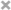# Xiuchuan Zhang

Personal Website

This is Xiuchuan's personal website.
I plan to post some of my current learning and review notes on it.
If you have any questions or suggestions, welcome to comment in my posts.

R编程 初学者 代码整理
Collecting of R Code (Noob)

## R Packages

install commands like install.packages and install_github
do library function to load them

• dplyr: a grammar of data manipulation
• ggplot2: for data visualization

### Basic

• Load Data: data() from data files or load()
• Dimensions of Data: dim(data_file_name)
• Names of variables: names(data_file_name)
• Single column of data: data_file_name\$variable_name
• Data structure: str(data_file_name)
• Create new data from old: new_data_file_name <- data_file_name
• Descending order: desc
• If else: ifelse(test, yes, no)
• Counting streak lengths: calc_streak(data_file_name)
• Table: table(data)
• Search the names for a fragment of the name: grep("search_name", names(data_file_name), value = TRUE)

### dplyr

dplyr

• data_file_name %>%
• group_by(variable_name) %>%:grouped the data by origin
• mutate(): adds new variables that are functions of existing variables
  data_file_name <- data_file_name %>%
mutate(new_variable = variable_A simple_mathematical_operator variable_B)

• select(): picks variables based on their names.
• filter(): picks cases based on their values.
  new_data_file_name <- data_file_name %>%
filter(variable_name logical_operator filter_condition)

• find the one is na: filter(is.na(variable_name))
• summarise(): reduces multiple values down to a single summary.
  data_file_name %>%
summarise(mean_variable = mean(variable_name), sd_variable = sd(variable_name), n = n())

• arrange(): changes the ordering of the rows.
  data_file_name %>%
summarise(median_variable = median(variable_name)) %>%
arrange(desc(median_variable))

• desc: Descending order
• distinct(): select distinct/unique rows
• sample(): simulation, uses random numbers to generate an outcome
  data_outcomes <- c("variable_outcome_A", "variable_outcome_B")
sample(data_outcomes, size = 100, replace = TRUE, prob = c( #variable_A_prob, #variable_B_prob ))


### ggplot2

• Making graphics:
  ggplot(data = data_file_name, aes(x = x_aes_name, y = y_aes_name)) +
geom_line() +
geom_point()

• Making side-by-side box plots: geom_boxplot()

• Making segmented bar plot:
  ggplot(data = data_file_name, aes(x = x_aes_name, fill = variable_name)) +
geom_bar()

• Making histogram:
  ggplot(data = data_file_name, aes(x = x_aes_name)) +
geom_histogram(binwidth = #number )


Support